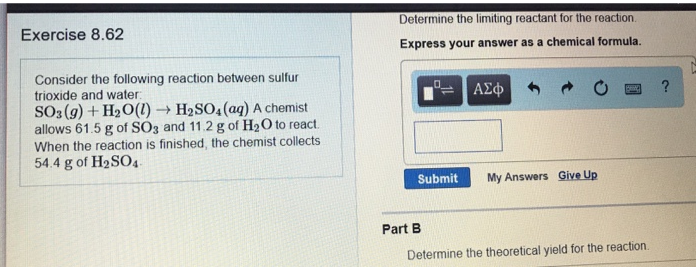# Problem: Consider the following reaction between sulfur trioxide and water: SO3 (g) + H2O (l) → H2SO4 (aq) A chemist allows 61.5 g of SO3 and 11.2 g of H2O to react When the reaction is finished: the chemist collects 54.4 g of H2SO4. Part ADetermine the limiting reactant for the reaction. Express your answer as a chemical formula. Part BDetermine the theoretical yield for the reaction.

###### FREE Expert Solution
88% (137 ratings)###### Problem Details

Consider the following reaction between sulfur trioxide and water:

SO3 (g) + H2O (l) → H2SO4 (aq)

A chemist allows 61.5 g of SO3 and 11.2 g of H2O to react When the reaction is finished: the chemist collects 54.4 g of H2SO4

Part A

Determine the limiting reactant for the reaction.

Part B

Determine the theoretical yield for the reaction.